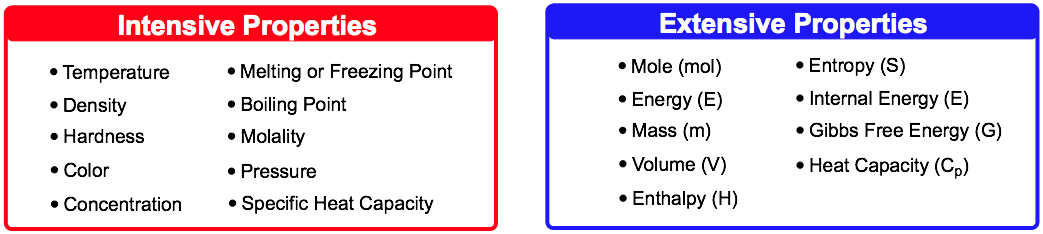Clutch Prep is now a part of Pearson
Ch.1 Matter and MeasurementsWorksheetSee all chapters

# Intensive vs. Extensive Properties

See all sections
Sections
What is Chemistry?
The Scientific Method
Classification of Matter
States of Matter
Physical & Chemical Changes
Chemical Properties
Physical Properties
Intensive vs. Extensive Properties
Temperature (Simplified)
Scientific Notation
SI Units (Simplified)
Metric Prefixes
Significant Figures (Simplified)
Significant Figures: Precision in Measurements
Significant Figures: In Calculations
Conversion Factors (Simplified)
Dimensional Analysis
Density
Specific Gravity
Density of Geometric Objects
Density of Non-Geometric Objects

Intensive Properties are independent and Extensive Properties are dependent on the size or amount of substance present

###### Understanding Intensive Properties

Concept #1: Intensive Properties

Example #1: Which of the following are examples of intensive properties?

i) Mass          ii) Length          iii) Melting point          iv) Volume          v) Luster

a) i, ii, & iv          b) ii & iii          c) ii, iii & v          d) iii & v

Practice: Which of the following is not an example of an intensive property?

Concept #2: Extensive PropertiesExample #2: Which of the following is classified as an extensive property?

a) Chemical Energy

b) Electrical Conductivity

c) Luster

d) Freezing Point

Practice: Which of the following is not an example of an extensive property?

Practice: Which of the following is an extensive property of a nitrogen molecule?